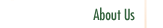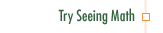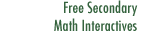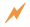Proportional Reasoning

Proportional Reasoning prepares the transition from a primary focus on arithmetic and skills with algorithms (typical of elementary and middle school) to a focus on algebra, where students use multiplicative, as well as additive, thinking.

Professional Development Goals

• Explore the relationship between proportional reasoning and algebraic thinking.
• Understand that students who appear to reason proportionally may in fact be following a procedure without understanding it.
• Understand methods for developing students' proportional reasoning.Click to Get Seeing Math™ SecondaryCourse Listing Proportional Reasoning Linear Functions Transformations of Linear Functions Linear Equations Systems of Linear Equations Quadratic Functions Transformations of Quadratic Functions Quadratic Equations Data Analysis